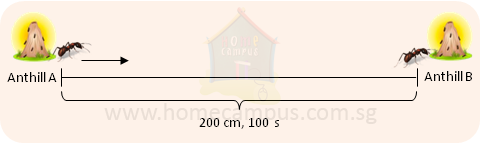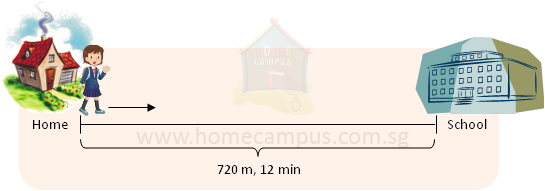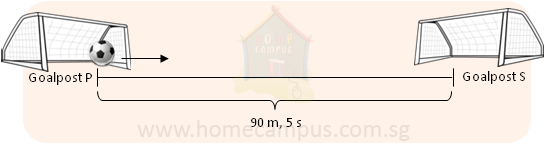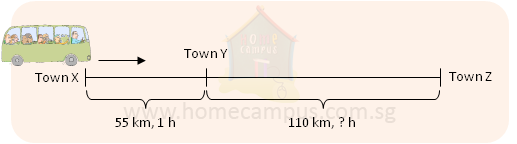## Distance, Time and Speed

Practice Unlimited Questions

#### What is Speed?

Speed describes how fast an object is moving. In other words, speed tells us the distance an object travels per unit of time.

The various units of speed are:
• cm/s (read as, centimetres per second)
• m/min (read as, metres per minute)
• m/s (read as, metres per second)
• km/h (read as, kilometres per hour)

#### Important formulas:

Speed  =
 Distance travelled Time taken

Distance travelled  =  Speed  ×  Time taken

Time taken  =
 Distance travelled Speed

#### 1. The ant below crawls from Anthill A to Anthill B in 100 seconds. Find its speed.The ant crawls 200 cm in 100 seconds.
In 1 s, the ant crawls 2 cm.
The ant's crawling speed is 2 cm/s.

#### 2. The girl below walks from home to school in 12 minutes. Find its speed.The girl walks 720 m in 12 minutes.
The girl walks 60 m in 1 min.
The girl's walking speed is 60 m/min.

#### 3. The soccer ball travels from Goalpost P to Goalpost S in 5 seconds. find its speed.The ball travels 90 m in 5 s.
In 1 s, the ball travels 18 m.
The ball travels at an speed of 18 m/s.

#### 4. The bus below travels from Town X to Town Y in 1 hour. a)   At this speed, how long will it take to reach Town Z from Town Y? b)   At this speed, how far will it be     1 2 hour after it leaves Town Z?In 1 hour, the bus travels 55 km.
The bus is travelling at a speed of 55 km/h.

a)    55 km    -->   1 h
110 km   -->   2 h
The bus will take 2 hours to reach Town Z from Town Y.

b)    1 h    -->   55 km

 1 2
h   -->
 55 2
km  =  27.5 km
The bus will be 27.5 km away
 1 2
h after it leaves Town Z.

#### 5. A bird flies in search of food from her nest in a tree to a stream 1800 metres away in 3 minutes. What is the speed at which she flies?

Distance travelled  =  1800 m

Time taken  =  3 min

Speed  =
 1800 m 3 min
=  600 m/min

The bird flies at a speed of 600 m/min.

#### 6. A lorry is going at a speed of 40 km/h. At this speed, how far can it go in     1 2 h?

Speed  =  40 km/h

Time taken  =
 1 2
h

Distance travelled  =  40 km/h  ×
 1 2
h  =  20 km

It can go 20 km in
 1 2
h.

#### 7. A train is running at a speed of 180 km/h. At this speed, how long will the train take to travel the 270-km long distance between Station A and Station B?

Speed  =  180 km/h

Distance travelled  =  270 km

Time taken  =
 270 km 180 km/h
=
 3 2
h  =
 1 1 2
h

The train will take
 1 1 2
h
to travel the distance between Station A and Station B.